# C# Lambda表达式的前世今生

1. 定义一个委托，包含指定的参数类型和返回值类型。
2. 在需要接收函数参数的方法中，使用该委托类型定义方法的参数签名。
3. 为指定的被传递的函数创建一个委托实例。

• Action ：无输入参数，无返回值
• Action ：支持1-16个输入参数，无返回值
• Func ：支持1-16个输入参数，有返回值

Action 委托返回 void 类型，Func 委托返回指定类型的值。通过使用这两种委托，在绝大多数情况下，上述的步骤 1 可以省略了。但是步骤 2 仍然是必需的，但仅是需要使用 Action 和 Func。

```Func<double, double> square = delegate(double x)
{
return x * x;
};```

```// The compiler cannot resolve this, which makes the usage of var impossible!
// Therefore we need to specify the type.
Action dummyLambda = () =>
{
Console.WriteLine("Hello World from a Lambda expression!");
};

// Can be used as with double y = square(25);
Func<double, double> square = x => x * x;

// Can be used as with double z = product(9, 5);
Func<double, double, double> product = (x, y) => x * y;

// Can be used as with printProduct(9, 5);
Action<double, double> printProduct = (x, y) => { Console.WriteLine(x * y); };

// Can be used as with
// var sum = dotProduct(new double[] { 1, 2, 3 }, new double[] { 4, 5, 6 });
Func<double[], double[], double> dotProduct = (x, y) =>
{
var dim = Math.Min(x.Length, y.Length);
var sum = 0.0;
for (var i = 0; i != dim; i++)
sum += x[i] + y[i];
return sum;
};

// Can be used as with var result = matrixVectorProductAsync(...);
Func<double[,], double[], Task<double[]>> matrixVectorProductAsync =
async (x, y) =>
{
var sum = 0.0;
/* do some stuff using await ... */
return sum;
};```

• 如果仅有一个入参，则可省略圆括号。
• 如果仅有一行语句，并且在该语句中返回，则可省略大括号，并且也可以省略 return 关键字。
• 通过使用 async 关键字，可以将 Lambda 表达式声明为异步执行。
• 大多数情况下，var 声明可能无法使用，仅在一些特殊的情况下可以使用。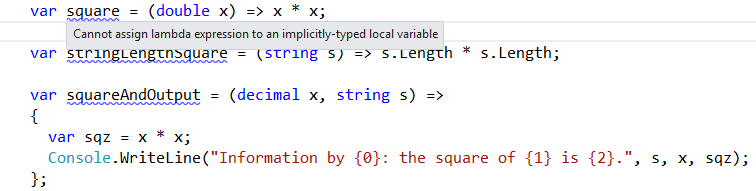```var a = 5;
Funcint, int> multiplyWith = x => x * a;

var result1 = multiplyWith(10); // 50
a = 10;
var result2 = multiplyWith(10); // 100```

```  static void DoSomeStuff()
{
var coeff = 10;
Funcint, int> compute = x => coeff * x;
Action modifier = () =>
{
coeff = 5;
};

var result1 = DoMoreStuff(compute); // 50

ModifyStuff(modifier);

var result2 = DoMoreStuff(compute); // 25
}

static int DoMoreStuff(Funcint, int> computer)
{
return computer(5);
}

static void ModifyStuff(Action modifier)
{
modifier();
}```

```var buttons = new Button;

for (var i = 0; i < buttons.Length; i++)
{
var button = new Button();
button.Text = (i + 1) + ". Button - Click for Index!";
button.OnClick += (s, e) => { Messagebox.Show(i.ToString()); };
buttons[i] = button;
}

//What happens if we click ANY button?!```

```var button = new Button();
var index = i;
button.Text = (i + 1) + ". Button - Click for Index!";
button.OnClick += (s, e) => { Messagebox.Show(index.ToString()); };
buttons[i] = button;```

## 表达式树

1. 我们传递的变量的名字是什么？
2. 我们使用的表达式体的结构是什么？
3. 在表达式体内我们用了哪些类型？

```Expressionint>> expr = model => model.MyProperty;
var member = expr.Body as MemberExpression;
var propertyName = memberExpression.Member.Name; //only execute if member != null```

```string WhatsMyName([CallerMemberName] string callingName = null)
{
return callingName;
}```

## Lambda 表达式的性能

```class StandardBenchmark : Benchmark
{
static double[] A;
static double[] B;

public static void Test()
{
var me = new StandardBenchmark();

Init();

for (var i = 0; i 10; i++)
{
var lambda = LambdaBenchmark();
var normal = NormalBenchmark();
}

me.PrintTable();
}

static void Init()
{
var r = new Random();
A = new double[LENGTH];
B = new double[LENGTH];

for (var i = 0; i )
{
A[i] = r.NextDouble();
B[i] = r.NextDouble();
}
}

static long LambdaBenchmark()
{
Funcdouble> Perform = () =>
{
var sum = 0.0;

for (var i = 0; i )
sum += A[i] * B[i];

return sum;
};
var iterations = new double;
var timing = new Stopwatch();
timing.Start();

for (var j = 0; j )
iterations[j] = Perform();

timing.Stop();
Console.WriteLine("Time for Lambda-Benchmark: t {0}ms",
timing.ElapsedMilliseconds);
return timing.ElapsedMilliseconds;
}

static long NormalBenchmark()
{
var iterations = new double;
var timing = new Stopwatch();
timing.Start();

for (var j = 0; j )
iterations[j] = NormalPerform();

timing.Stop();
Console.WriteLine("Time for Normal-Benchmark: t {0}ms",
timing.ElapsedMilliseconds);
return timing.ElapsedMilliseconds;
}

static double NormalPerform()
{
var sum = 0.0;

for (var i = 0; i )
sum += A[i] * B[i];

return sum;
}
}```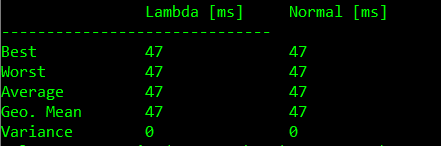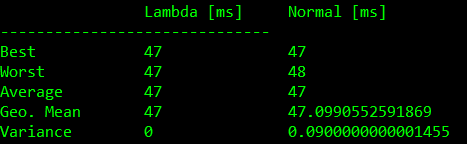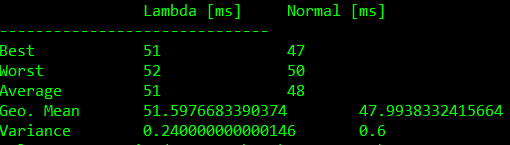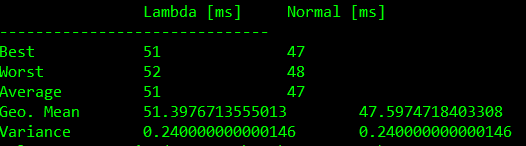Test Lambda [ms] Normal [ms] 0 45+-1 46+-1 1 44+-1 46+-2 2 49+-3 45+-2 3 48+-2 45+-2## MSIL揭秘Lambda表达式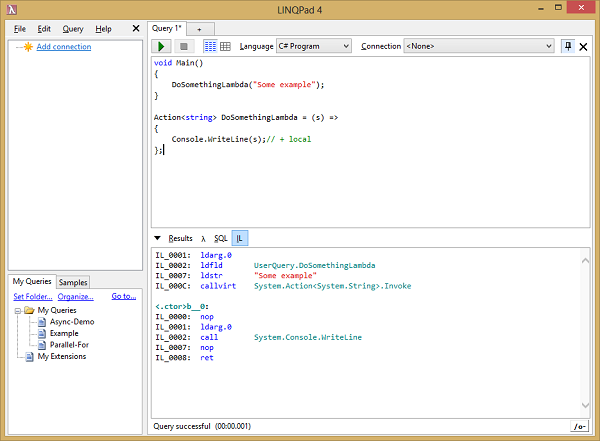```void Main()
{
DoSomethingLambda("some example");
DoSomethingNormal("some example");
}```

Lambda 表达式：

```Actionstring> DoSomethingLambda = (s) =>
{
Console.WriteLine(s);// + local
};```

```void DoSomethingNormal(string s)
{
Console.WriteLine(s);
}```

```  IL_0001:  ldarg.0
IL_0002:  ldfld       UserQuery.DoSomethingLambda
IL_0007:  ldstr       "some example"
IL_000C:  callvirt    System.Action.Invoke
IL_0011:  nop
IL_0012:  ldarg.0
IL_0013:  ldstr       "some example"
IL_0018:  call        UserQuery.DoSomethingNormal

DoSomethingNormal:
IL_0000:  nop
IL_0001:  ldarg.1
IL_0002:  call        System.Console.WriteLine
IL_0007:  nop
IL_0008:  ret

b__0:
IL_0000:  nop
IL_0001:  ldarg.0
IL_0002:  call        System.Console.WriteLine
IL_0007:  nop
IL_0008:  ret```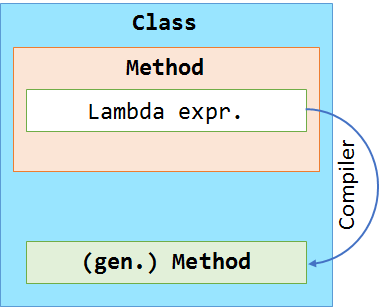``` void Main()
{
int local = 5;

Actionstring> DoSomethingLambda = (s) => {
Console.WriteLine(s + local);
};

global = local;

DoSomethingLambda("Test 1");
DoSomethingNormal("Test 2");
}

int global;

void DoSomethingNormal(string s)
{
Console.WriteLine(s + global);
}```

```  IL_0000:  newobj      UserQuery+c__DisplayClass1..ctor
IL_0005:  stloc.1     // CS\$8__locals2
IL_0006:  nop
IL_0007:  ldloc.1     // CS\$8__locals2
IL_0008:  ldc.i4.5
IL_0009:  stfld       UserQuery+c__DisplayClass1.local
IL_000E:  ldloc.1     // CS\$8__locals2
IL_000F:  ldftn       UserQuery+c__DisplayClass1.b__0
IL_0015:  newobj      System.Action..ctor
IL_001A:  stloc.0     // DoSomethingLambda
IL_001B:  ldarg.0
IL_001C:  ldloc.1     // CS\$8__locals2
IL_001D:  ldfld       UserQuery+c__DisplayClass1.local
IL_0022:  stfld       UserQuery.global
IL_0027:  ldloc.0     // DoSomethingLambda
IL_0028:  ldstr       "Test 1"
IL_002D:  callvirt    System.Action.Invoke
IL_0032:  nop
IL_0033:  ldarg.0
IL_0034:  ldstr       "Test 2"
IL_0039:  call        UserQuery.DoSomethingNormal
IL_003E:  nop

DoSomethingNormal:
IL_0000:  nop
IL_0001:  ldarg.1
IL_0002:  ldarg.0
IL_0003:  ldfld       UserQuery.global
IL_0008:  box         System.Int32
IL_000D:  call        System.String.Concat
IL_0012:  call        System.Console.WriteLine
IL_0017:  nop
IL_0018:  ret

c__DisplayClass1.b__0:
IL_0000:  nop
IL_0001:  ldarg.1
IL_0002:  ldarg.0
IL_0003:  ldfld       UserQuery+c__DisplayClass1.local
IL_0008:  box         System.Int32
IL_000D:  call        System.String.Concat
IL_0012:  call        System.Console.WriteLine
IL_0017:  nop
IL_0018:  ret

c__DisplayClass1..ctor:
IL_0000:  ldarg.0
IL_0001:  call        System.Object..ctor
IL_0006:  ret```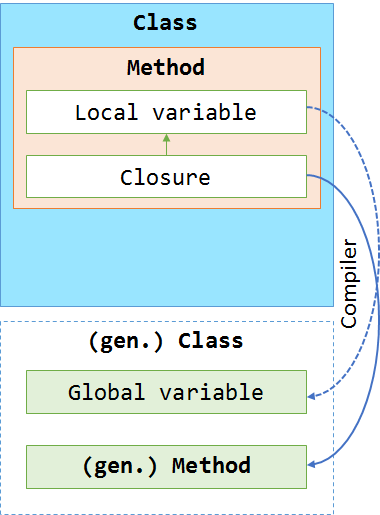(0)
0%

(0)
0%